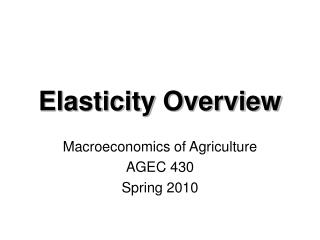Download PresentationElasticity Overview

# Elasticity Overview - PowerPoint PPT PresentationDownload Presentation## Elasticity Overview

- - - - - - - - - - - - - - - - - - - - - - - - - - - E N D - - - - - - - - - - - - - - - - - - - - - - - - - - -
##### Presentation Transcript

1. Elasticity Overview Macroeconomics of Agriculture AGEC 430 Spring 2010

2. D D S S Market Equilibrium Price S D Initial market equilibrium Pe Quantity Qe

3. Demand/Supply Model Demand equation: Qd = a0 - a1(Price) +  ai (demand shifters) Supply equation: Qs = b0 +b1(price) +  bi (supply shifters) Market equilibrium: Qd = Qs To forecast price, substitute demand and supply definitions into the equation for market equilibrium and solve for price.

4. Concept of Elasticity Demand elasticities Own price elasticity = %Q/ %P Income elasticity = %Q/ %Y Cross Price elasticity = %Q/ %Px Related concept Price flexibility = %P/ %Q

5. Elastic Demand Curve Price c Pb Drop in price Brings about a larger increase in the quantity demanded Pa 0 Qb Qa Quantity

6. Elastic Demand Curve Price Producer revenue increases since %P is less that %Q. Revenue before the change was 0PbaQb. Revenue after the change was 0PabQa. c a Pb b Pa 0 Qb Qa Quantity

7. Elastic Demand Curve Price Producer revenue increases since %P is less that %Q. Revenue before the change was 0PbaQb. Revenue after the change was 0PabQa. c a Pb b Pa 0 Qb Qa Quantity

8. Elastic Demand Curve Price Producer revenue increases since %P is less that %Q. Revenue before the change was 0PbaQb. Revenue after the change was 0PabQa. c a Pb b Pa 0 Qb Qa Quantity

9. Inelastic Demand Curve Price Pb Drop in price Pa Brings about a smaller increase in the quantity demanded Qb Qa Quantity

10. Inelastic Demand Curve Price a Producer revenue falls since %P is greater than %Q. Revenue before the change was 0PbaQb. Revenue after the change was 0PabQa. Pb b Pa 0 Qb Qa Quantity

11. Inelastic Demand Curve Price a Producer revenue falls since %P is greater than %Q. Revenue before the change was 0PbaQb. Revenue after the change was 0PabQa. Pb b Pa 0 Qb Qa Quantity

12. Revenue Implications

13. Revenue Implications